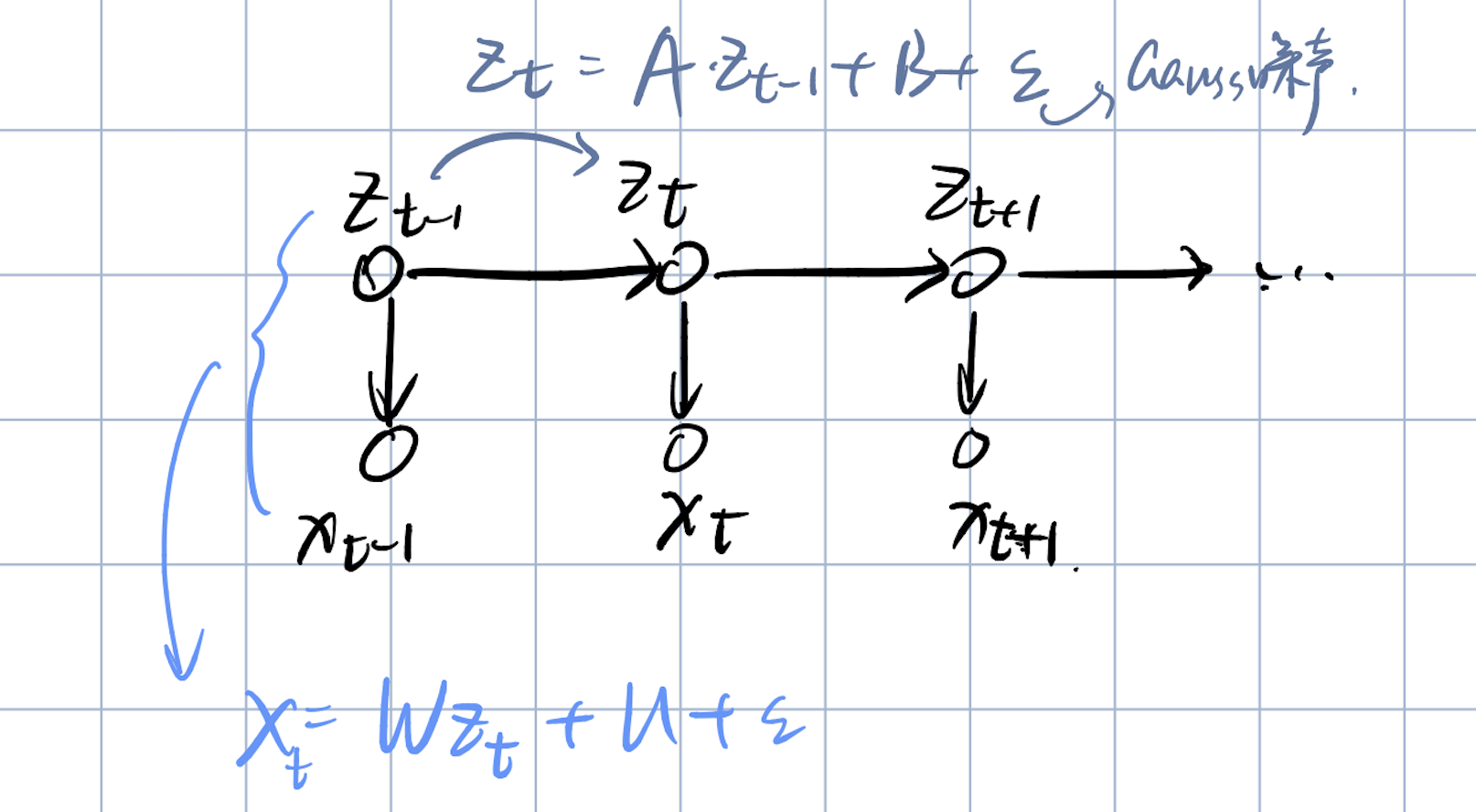# 「Machine Learning-1」Math Basics

## Math basics(probability and mathematical statistics) for machine learning

Posted by Culaccino on April 8, 2020

——————

# 概率论补充

#### 1)高斯分布（正态分布）ps:这种线性高斯模型在P-PCA降维中非常常用，具体可以见后序博客。

#### 2)求边缘概率及条件概率

①求$P(X_a)$： $% $

$X_a\sim N(\mu_a,\sigma_{aa})$

②求 $P(X_b|X_a):$

#### 3)求联合概率分布

①求$P(y)$:

②求 $P(x|y):$

$% $